# ML Aggarwal Solutions for Class 6 Maths Chapter 15 Data Handling

ML Aggarwal Solutions for Class 6 Maths Chapter 15 Data Handling provides the technique of handling the given set of data, with illustrations. The solutions are prepared by expert teachers, with the aim of boosting exam preparation among students. Those who find obstacles in solving textbook problems, are suggested to practice ML Aggarwal Solutions on a regular basis. For more conceptual knowledge, students can make use of ML Aggarwal Solutions for Class 6 Maths Chapter 15 Data Handling PDF, with the help of the links available below.

Chapter 15 mainly deals with the study of a given set of data in the form of frequency distribution table, pictograph and bar graph. The solutions are prepared in an interactive manner to help students clear their doubts quickly. These solutions also help them to improve their time management skills, along with problem solving skills, which are vital from the exam perspective.

## ML Aggarwal Solutions for Class 6 Maths Chapter 15 Data Handling Download PDF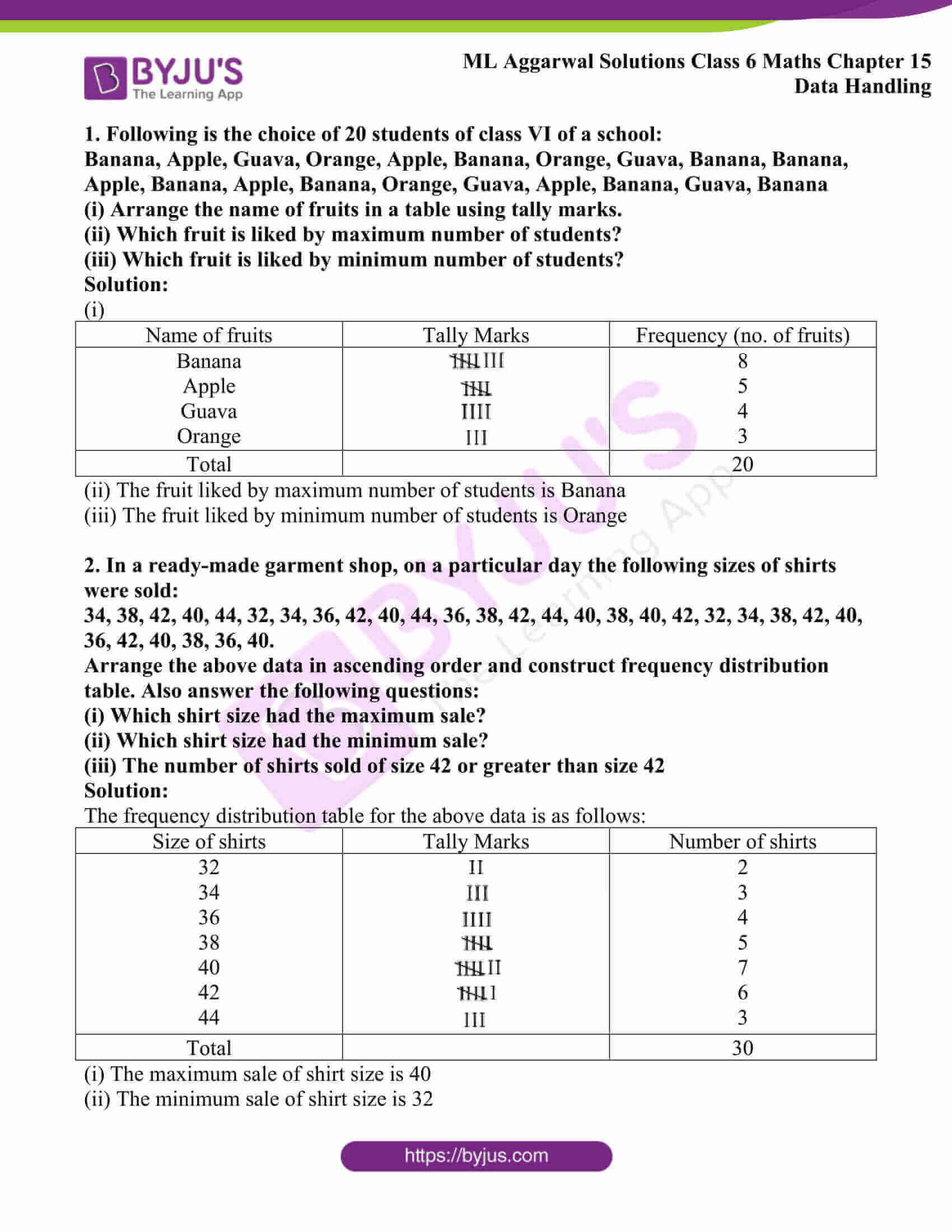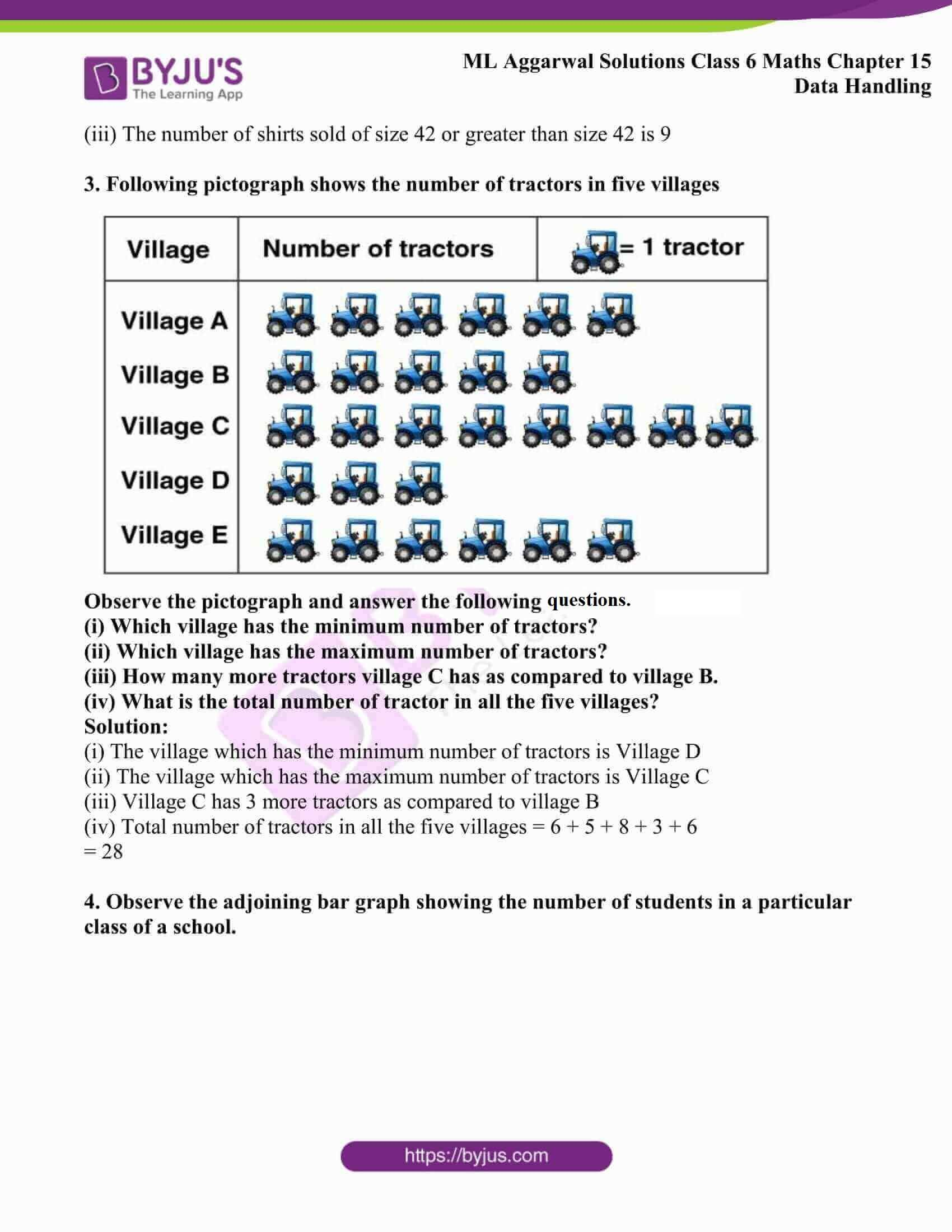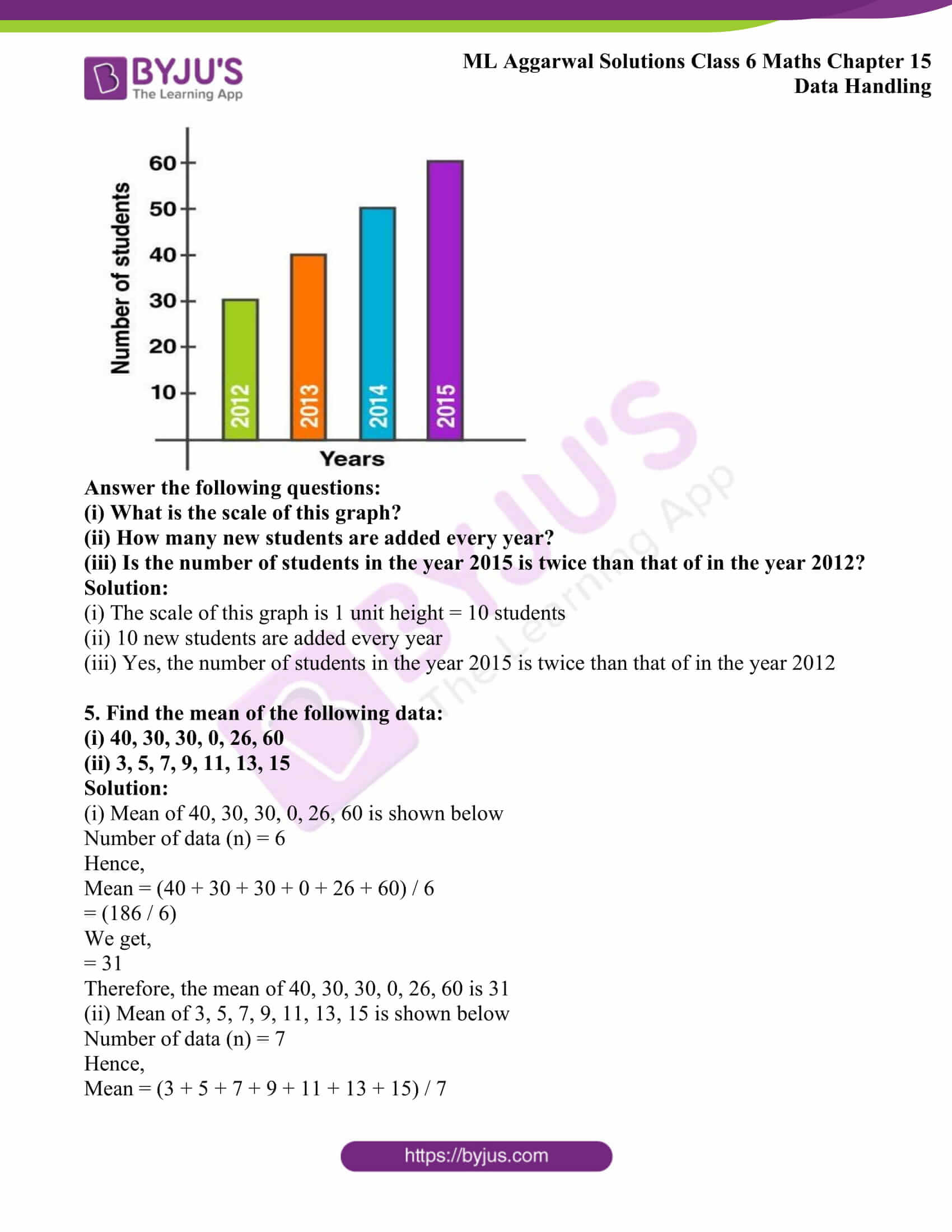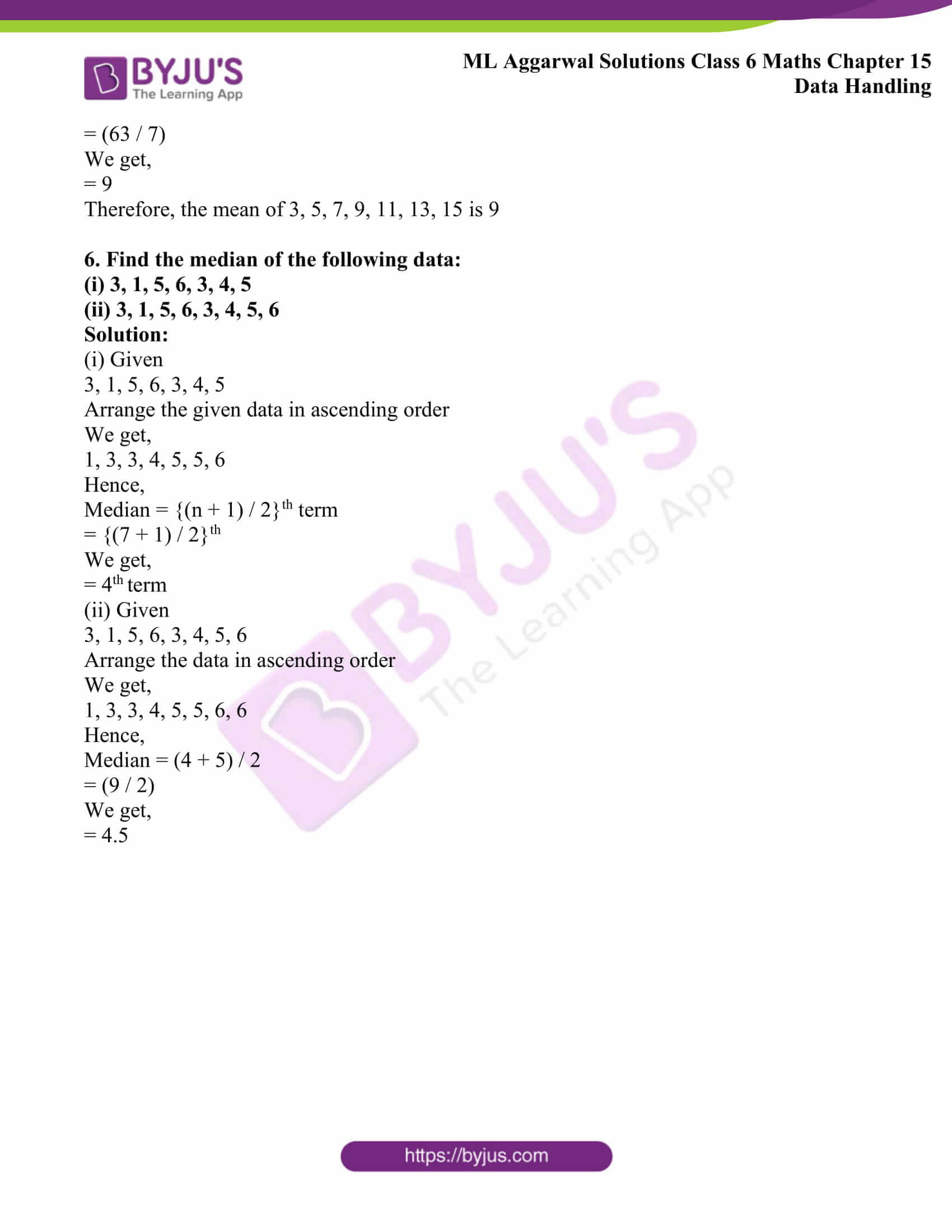## Access ML Aggarwal Solutions for Class 6 Maths Chapter 15 Data Handling

1. Following is the choice of 20 students of class VI of a school:

Banana, Apple, Guava, Orange, Apple, Banana, Orange, Guava, Banana, Banana, Apple, Banana, Apple, Banana, Orange, Guava, Apple, Banana, Guava, Banana

(i) Arrange the name of fruits in a table using tally marks.

(ii) Which fruit is liked by maximum number of students?

(iii) Which fruit is liked by minimum number of students?

Solution:

(i)

 Name of fruits Tally Marks Frequency (no. of fruits) Banana Apple Guava Orange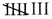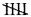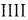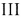8 5 4 3 Total 20

(ii) The fruit liked by maximum number of students is Banana

(iii) The fruit liked by minimum number of students is Orange

2. In a ready-made garment shop, on a particular day the following sizes of shirts were sold:

34, 38, 42, 40, 44, 32, 34, 36, 42, 40, 44, 36, 38, 42, 44, 40, 38, 40, 42, 32, 34, 38, 42, 40, 36, 42, 40, 38, 36, 40.

Arrange the above data in ascending order and construct frequency distribution table. Also answer the following questions:

(i) Which shirt size had the maximum sale?

(ii) Which shirt size had the minimum sale?

(iii) The number of shirts sold of size 42 or greater than size 42

Solution:

The frequency distribution table for the above data is as follows:

 Size of shirts Tally Marks Number of shirts 32 34 36 38 40 42 44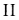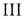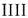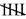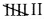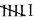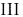2 3 4 5 7 6 3 Total 30

(i) The maximum sale of shirt size is 40

(ii) The minimum sale of shirt size is 32

(iii) The number of shirts sold of size 42 or greater than size 42 is 9

3. Following pictograph shows the number of tractors in five villages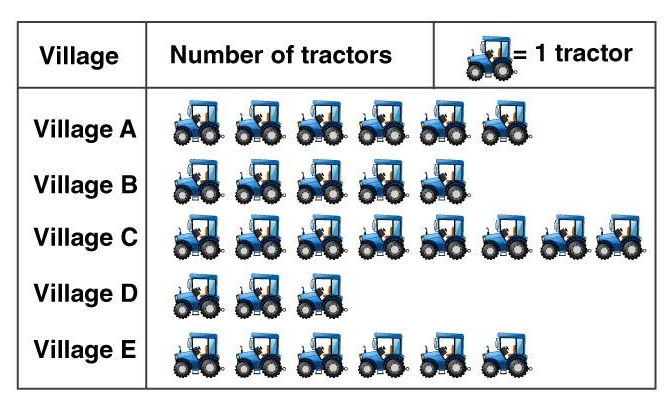Observe the pictograph and answer the following questions.

(i) Which village has the minimum number of tractors?

(ii) Which village has the maximum number of tractors?

(iii) How many more tractors village C has as compared to village B.

(iv) What is the total number of tractor in all the five villages?

Solution:

(i) The village which has the minimum number of tractors is Village D

(ii) The village which has the maximum number of tractors is Village C

(iii) Village C has 3 more tractors as compared to village B

(iv) Total number of tractors in all the five villages = 6 + 5 + 8 + 3 + 6

= 28

4. Observe the adjoining bar graph showing the number of students in a particular class of a school.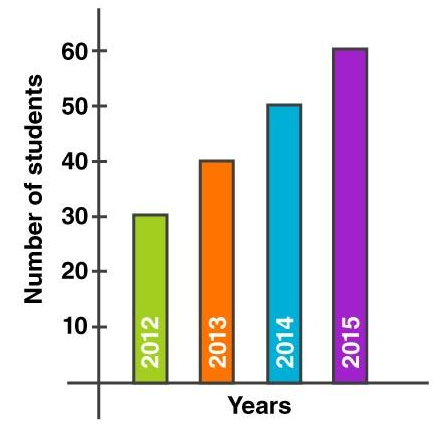(i) What is the scale of this graph?

(ii) How many new students are added every year?

(iii) Is the number of students in the year 2015 is twice than that of in the year 2012?

Solution:

(i) The scale of this graph is 1 unit height = 10 students

(ii) 10 new students are added every year

(iii) Yes, the number of students in the year 2015 is twice than that of in the year 2012

5. Find the mean of the following data:

(i) 40, 30, 30, 0, 26, 60

(ii) 3, 5, 7, 9, 11, 13, 15

Solution:

(i) Mean of 40, 30, 30, 0, 26, 60 is shown below

Number of data (n) = 6

Hence,

Mean = (40 + 30 + 30 + 0 + 26 + 60) / 6

= (186 / 6)

We get,

= 31

Therefore, the mean of 40, 30, 30, 0, 26, 60 is 31

(ii) Mean of 3, 5, 7, 9, 11, 13, 15 is shown below

Number of data (n) = 7

Hence,

Mean = (3 + 5 + 7 + 9 + 11 + 13 + 15) / 7

= (63 / 7)

We get,

= 9

Therefore, the mean of 3, 5, 7, 9, 11, 13, 15 is 9

6. Find the median of the following data:

(i) 3, 1, 5, 6, 3, 4, 5

(ii) 3, 1, 5, 6, 3, 4, 5, 6

Solution:

(i) Given

3, 1, 5, 6, 3, 4, 5

Arrange the given data in ascending order

We get,

1, 3, 3, 4, 5, 5, 6

Hence,

Median = {(n + 1) / 2}th term

= {(7 + 1) / 2}th

We get,

= 4th term

(ii) Given

3, 1, 5, 6, 3, 4, 5, 6

Arrange the data in ascending order

We get,

1, 3, 3, 4, 5, 5, 6, 6

Hence,

Median = (4 + 5) / 2

= (9 / 2)

We get,

= 4.5